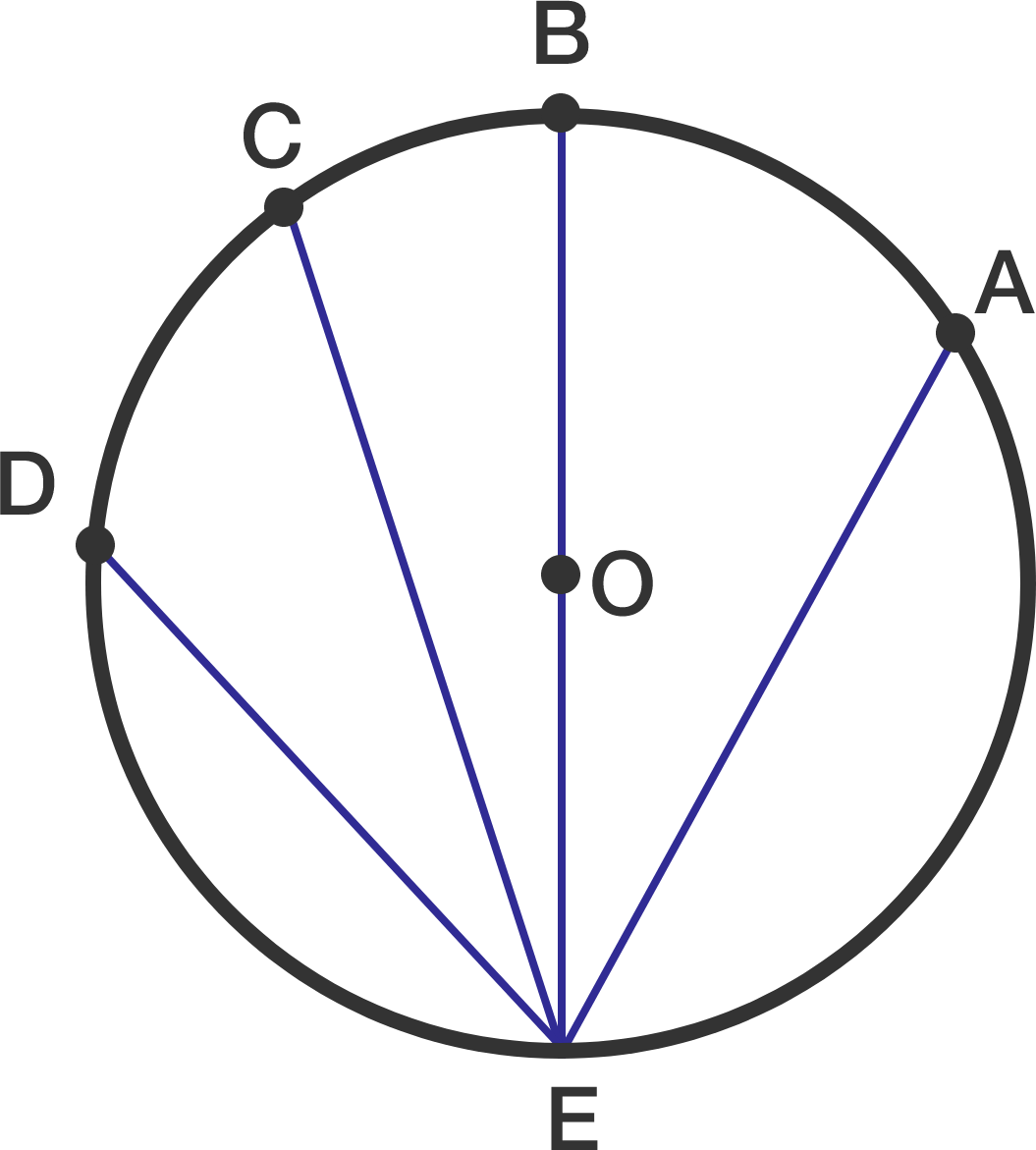# Which will win the race?As the image shows, $O$ is the center of a circle, $E$ is at the bottom of the circle, and $AE,BE,CE,DE$ are all smooth inclines along the circle.

Now, let's put four balls at rest at points $A,B,C,D.$

If whichever gets to point $E$ first wins the race, ignoring all the frictions, which ball will win the race?

×